Test: Progression (AP And GP)- 4

# Test: Progression (AP And GP)- 4

Test Description

## 15 Questions MCQ Test Quantitative Aptitude (Quant) | Test: Progression (AP And GP)- 4

Test: Progression (AP And GP)- 4 for Banking Exams 2023 is part of Quantitative Aptitude (Quant) preparation. The Test: Progression (AP And GP)- 4 questions and answers have been prepared according to the Banking Exams exam syllabus.The Test: Progression (AP And GP)- 4 MCQs are made for Banking Exams 2023 Exam. Find important definitions, questions, notes, meanings, examples, exercises, MCQs and online tests for Test: Progression (AP And GP)- 4 below.
Solutions of Test: Progression (AP And GP)- 4 questions in English are available as part of our Quantitative Aptitude (Quant) for Banking Exams & Test: Progression (AP And GP)- 4 solutions in Hindi for Quantitative Aptitude (Quant) course. Download more important topics, notes, lectures and mock test series for Banking Exams Exam by signing up for free. Attempt Test: Progression (AP And GP)- 4 | 15 questions in 15 minutes | Mock test for Banking Exams preparation | Free important questions MCQ to study Quantitative Aptitude (Quant) for Banking Exams Exam | Download free PDF with solutions
 1 Crore+ students have signed up on EduRev. Have you?
Test: Progression (AP And GP)- 4 - Question 1

### How many terms are there in the AP 20, 25, 30,… 130.

Detailed Solution for Test: Progression (AP And GP)- 4 - Question 1

In order to count the number of terms in the AP, use the short cut:
[(last term – first term)/ common difference] + 1. In this case it would become:
[(130 – 20)/5] +1 = 23. Option (b) is correct.

Test: Progression (AP And GP)- 4 - Question 2

### Find the 1st term of an AP whose 8th and 12th terms are respectively 39 and 59.

Detailed Solution for Test: Progression (AP And GP)- 4 - Question 2

Since the 8th and the 12th terms of the AP are given as 39 and 59 respectively, the difference
between the two terms would equal 4 times the common difference. Thus we get 4d = 59 – 39 =
20. This gives us d = 5. Also, the 8th term in the AP is represented by a + 7d, we get:
a + 7d = 39 Æ a + 7 × 5 = 39 Æ a = 4. Option (c) is correct.

Test: Progression (AP And GP)- 4 - Question 3

### There is an AP 1, 3, 5…. Which term of this AP is 55?

Detailed Solution for Test: Progression (AP And GP)- 4 - Question 3

The number of terms in a series are found by: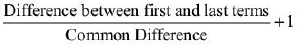Test: Progression (AP And GP)- 4 - Question 4

Find the lowest number in an AP such that the sum of all the terms is 105 and greatest term is 6 times the least.

Detailed Solution for Test: Progression (AP And GP)- 4 - Question 4

Trying Option (a),
We get least term 5 and largest term 30 (since the largest term is 6 times the least term).
The average of the A.P becomes (5 + 30)/2 = 17.5
Thus, 17.5 × n = 105 gives us:
to get a total of 105 we need n = 6 i.e. 6 terms in this A.P. That means the A.P. should look like:
5, _ , _ , _, _, 30.
It can be easily seen that the common difference should be 5. The A.P, 5, 10, 15, 20, 25, 30 fits the
situation.
The same process used for option (b) gives us the A.P. 10, 35, 60. (10 + 35 + 60 = 105) and in the
third option 15, 90 (15 + 90 = 105).
Hence, all the three options are correct.

Test: Progression (AP And GP)- 4 - Question 5

A sum of money kept in a bank amounts to ` 1240 in 4 years and ` 1600 in 10 years at simpleInterest. Find the sum.

Detailed Solution for Test: Progression (AP And GP)- 4 - Question 5

The difference between the amounts at the end of 4 years and 10 years will be the simple interest
on the initial capital for 6 years.
Hence, 360/6 = 60 =(simple interest.)
Also, the Simple Interest for 4 years when added to the sum gives 1240 as the amount.
Hence, the original sum must be 1000.

Test: Progression (AP And GP)- 4 - Question 6

The sum of the first 16 terms of an AP whose first term and third term are 5 and 15 respectively is

Detailed Solution for Test: Progression (AP And GP)- 4 - Question 6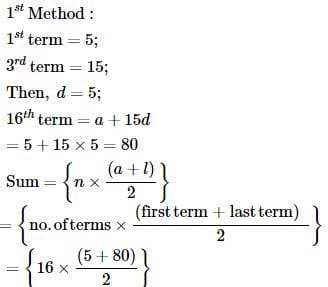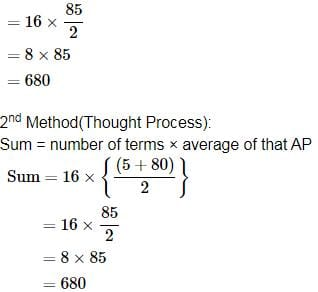Test: Progression (AP And GP)- 4 - Question 7

The least value of n for which the sum of the series 5 + 8 + 11… n terms is not less than 670 is

Detailed Solution for Test: Progression (AP And GP)- 4 - Question 7

Solve this question through trial and error by using values of n from the options:
For 19 terms, the series would be 5 + 8 + 11 + …. + 59 which would give us a sum for the series
as 19 × 32 = 608. The next term (20th term of the series) would be 62. Thus, 608 + 62 = 670
would be the sum to 20 terms. It can thus be concluded that for 20 terms the value of the sum of the
series is not less than 670. Option (a) is correct.

Test: Progression (AP And GP)- 4 - Question 8

How many terms are there in the GP 5, 20, 80, 320,… 20480?

Detailed Solution for Test: Progression (AP And GP)- 4 - Question 8

The series would be 5, 20, 80, 320, 1280, 5120, 20480. Thus, there are a total of 7 terms in the
series. Option (c) is correct.

Test: Progression (AP And GP)- 4 - Question 9

If the fifth term of a GP is 81 and first term is 16, what will be the 4th term of the GP?

Detailed Solution for Test: Progression (AP And GP)- 4 - Question 9

16r4 = 81 Æ r4 = 81/16 Æ r = 3/2. Thus, 4th term = ar3 = 16 × (3/2)3 = 54. Option (c) is correct

Test: Progression (AP And GP)- 4 - Question 10

The sum of three numbers in a GP is 14 and the sum of their squares is 84. Find the largestnumber.

Detailed Solution for Test: Progression (AP And GP)- 4 - Question 10

Visualising the squares below 84, we can see that the only way to get the sum of 3 squares as 84
is: 22 + 42 + 82 = 4 + 16 + 64. The largest number is 8. Option (a) is correct.

Test: Progression (AP And GP)- 4 - Question 11

How many natural numbers between 300 to 500 are multiples of 7?

Detailed Solution for Test: Progression (AP And GP)- 4 - Question 11

The series will be 301, 308, …….. 497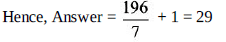Test: Progression (AP And GP)- 4 - Question 12

If a man saves ` 4 more each year than he did the year before and if he saves ` 20 in the first year,after how many years will his savings be more than ` 1000 altogether?

Detailed Solution for Test: Progression (AP And GP)- 4 - Question 12

We need the sum of the series 20 + 24 + 28 to cross 1000. Trying out the options, we can see that
in 20 years the sum of his savings would be: 20 + 24 + 28 + … + 96. The sum of this series would
be 20 × 58 =1160. If we remove the 20th year we will get the series for savings for 19 years. The
series would be 20 + 24 + 28 + …. 92. Sum of the series would be 1160 – 96 = 1064. If we
remove the 19th year’s savings the savings would be 1064 – 92 which would go below 1000.
Thus, after 19 years his savings would cross 1000. Option (a) is correct.

Test: Progression (AP And GP)- 4 - Question 13

The 4th and 10th term of an GP are 1/3 and 243 respectively. Find the 2nd term.

Detailed Solution for Test: Progression (AP And GP)- 4 - Question 13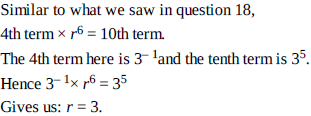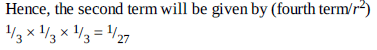[Note: To go forward in a G.P. you multiply by the common ratio, to go backward in a G.P. you
divide by the common ratio.]

Test: Progression (AP And GP)- 4 - Question 14

The sum of 5 numbers in AP is 30 and the sum of their squares is 220. Which of the following is the third term?

Detailed Solution for Test: Progression (AP And GP)- 4 - Question 14

Since the sum of 5 numbers in AP is 30, their average would be 6. The average of 5 terms in AP is
also equal to the value of the 3rd term (logic of the middle term of an AP). Hence, the third term’s
value would be 6. Option (b) is correct.

Test: Progression (AP And GP)- 4 - Question 15

The sum of the first four terms of an AP is 28 and sum of the first eight terms of the same AP is 88.Find the sum of the first 16 terms of the AP?

Detailed Solution for Test: Progression (AP And GP)- 4 - Question 15

Think like this:
The average of the first 4 terms is 7, while the average of the first 8 terms must be 11.
Now visualize this :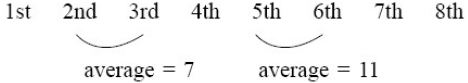Hence, d = 4/2 = 2 [Note: understand this as a property of an A.P.]
Hence, the average of the 6th and 7th terms = 15 and the average of the 8th and 9th term = 19
But this (19) also represents the average of the 16 term A.P.
Hence, required answer = 16 × 19 = 304.

## Quantitative Aptitude (Quant)

163 videos|163 docs|131 tests
 Use Code STAYHOME200 and get INR 200 additional OFF Use Coupon Code
Information about Test: Progression (AP And GP)- 4 Page
In this test you can find the Exam questions for Test: Progression (AP And GP)- 4 solved & explained in the simplest way possible. Besides giving Questions and answers for Test: Progression (AP And GP)- 4, EduRev gives you an ample number of Online tests for practice

## Quantitative Aptitude (Quant)

163 videos|163 docs|131 tests